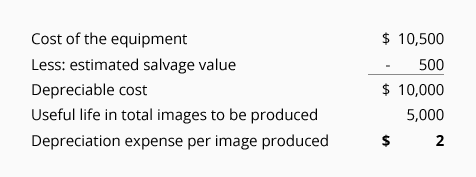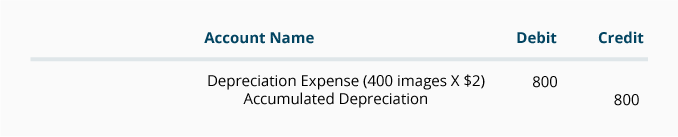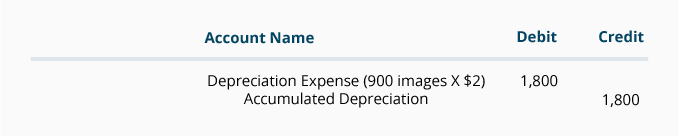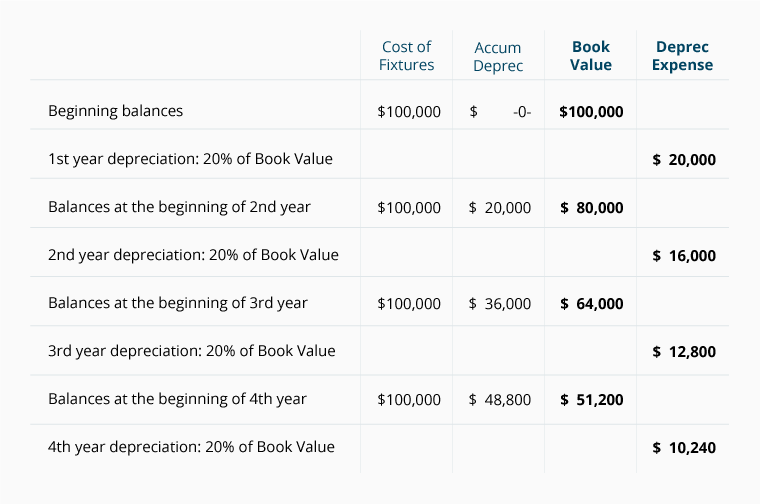## Units-of-Activity Depreciation

### Depreciation Not Based on Years

In most depreciation methods, an asset's estimated useful life is expressed in years. However, in the units-of-activity method (and in the similar units-of-production method), an asset's estimated useful life is expressed in units of output. In the units-of-activity method, the accounting period's depreciation expense is not a function of the passage of time. Instead, each accounting period's depreciation expense is based on the asset's usage during the accounting period.

### Examples of Units-of-Activity Depreciation

To introduce the concept of the units-of-activity method, let's assume that a service business purchases unique equipment at a cost of \$20,000. Over the equipment's useful life, the business estimates that the equipment will produce 5,000 valuable items. Assuming there is no salvage value for the equipment, the business will report \$4 (\$20,000/5,000 items) of depreciation expense for each item produced. If 80 items were produced during the first month of the equipment's use, the depreciation expense for the month will be \$320 (80 items X \$4). If in the next month only 10 items are produced by the equipment, only \$40 (10 items X \$4) of depreciation will be reported.

Now let's illustrate the units-of-activity method of depreciation by using a different example:

• On July 1, 2021, the company paid \$10,500 to purchase special equipment to produce elaborate images for its clients

• The company estimated that this equipment will have a useful life of 5,000 images

• The company estimated that the equipment will be sold for \$500 at the end of its useful life

Using the above information, the calculation of the units-of-activity method of depreciation begins with the following:Over the life of the equipment, the maximum total amount of depreciation expense is \$10,000. However, the amount of depreciation expense in any year depends on the number of images. Whether it's a partial year or a full year is not relevant.

The depreciation expense for any accounting period is calculated by multiplying the number of images produced times \$2 per image. For instance, if 400 images are produced from July 1 through December 31, 2021, the depreciation for 2021 will be recorded as follows:If 900 images are produced in the year 2022, the depreciation entry for 2022 will be recorded as follows:In this example, the depreciation will continue until the credit balance in Accumulated Depreciation reaches \$10,000 (the equipment's depreciable cost). If the equipment continues to be used, no further depreciation expense will be reported. The account balances remain in the general ledger until the equipment is sold, scrapped, etc.

## Double-Declining-Balance (DDB) Depreciation

### DDB is an Accelerated Method of Depreciation

The double-declining-balance (DDB) method, which is also referred to as the 200%-declining-balance method, is one of the accelerated methods of depreciation. DDB is an accelerated method because more depreciation expense is reported in the early years of an asset's useful life and less depreciation expense in the later years.

The "double" or "200%" means two times straight-line rate of depreciation. For instance, if an asset's estimated useful life is 10 years, the straight-line rate of depreciation is 10% (100% divided by 10 years) per year. Therefore, the "double" or "200%" will mean a depreciation rate of 20% per year.

The "declining-balance" refers to the asset's book value or carrying value (the asset's cost minus its accumulated depreciation). Recall that the asset's book value declines each time that depreciation is credited to the related contra asset account Accumulated Depreciation.

Therefore, the DDB depreciation calculation for an asset with a 10-year useful life will have a DDB depreciation rate of 20%. In the first accounting year that the asset is used, the 20% will be multiplied times the asset's cost since there is no accumulated depreciation. In the following accounting years, the 20% is multiplied times the asset's book value at the beginning of the accounting year. This differs from other depreciation methods where an asset's depreciable cost is used.

In DDB depreciation the asset's estimated salvage value is initially ignored in the calculations. However, the depreciation will stop when the asset's book value is equal to the estimated salvage value.

NOTE:
Although accelerated depreciation methods may more accurately coincide with the way some assets lose value, companies are reluctant to have their income statements show less net income and earnings per share than is required. As a result, companies are not interested in reporting larger depreciation expense in the early years of their assets' lives (and lower depreciation in future years).

However, when it comes to taxable income and the related income tax payments, it is a different story. In the U.S. companies are permitted to use straight-line depreciation on their income statements while using accelerated depreciation on their income tax returns. You can find more information on depreciation for income tax reporting at www.IRS.gov.

### Example of Double-Declining-Balance Depreciation

To illustrate the double-declining-balance method of depreciation, we will use the following information:

• A retailer purchased fixtures on January 1 at a cost of \$100,000

• The estimated useful life is 10 years (resulting in a straight-line depreciation rate of 10%)

• The DDB rate will be 20% (200% or double the straight-line rate of 10%)

• The estimated salvage value at the end of its useful life is \$8,000

Below is a table showing the first four years of the DDB depreciation:Note that the estimated salvage value of \$8,000 was not considered in calculating each year's depreciation expense. In our example, the depreciation expense will continue until the amount in Accumulated Depreciation reaches a credit balance of \$92,000 (cost of \$100,000 minus \$8,000 of salvage value).

[In practice, companies often assume \$0 salvage value and will switch from DDB to straight-line depreciation towards the end of the asset's useful life in order to fully depreciate the asset's cost.]

## Sum-of-the-Years'-Digits (SYD) Depreciation

### SYD is An Accelerated Method of Depreciation

The sum-of-the-years'-digits (SYD) depreciation method is also another form of accelerated depreciation since it results in more depreciation expense in the early years of the asset's useful life and less in the later years (as compared to the straight-line method).

The "sum-of-the-years'-digits" refers to adding the digits in the years of an asset's useful life. For example, if an asset has a useful life of 5 years, the sum of the digits 1 through 5 is equal to 15 (1 + 2 + 3 + 4 + 5).

An asset with a useful life of 10 years will have the following sum of its years' digits:

1 + 2 + 3 + 4 + 5 + 6 + 7 + 8 + 9 + 10 = 55

A fast way to compute the sum of the digits in the asset's useful life is to use this formula: n(n+1) divided by 2. If an asset's useful life is 10 years, then n = 10. The sum of the digits for an asset with a useful life of 10 years = 10(10+1)/2 = 10(11)/2 = 110/2 = 55.

In the case of an asset with a 10-year useful life, the depreciation expense in the first full year of the asset's life will be 10/55 times the asset's depreciable cost. The depreciation for the 2nd year will be 9/55 times the asset's depreciable cost. This pattern will continue and the depreciation for the 10th year will be 1/55 times the asset's depreciable cost.

### Example of Sum-of-the-Years'-Digits Depreciation

Now we will use the following information to calculate the SYD depreciation:

• A retailer purchased fixtures on January 1 at a cost of \$115,000

• The estimated useful life is 10 years

• The estimated salvage value at the end of its useful life is \$5,000

• The depreciable cost of the fixtures is \$110,000 (cost of \$115,000 minus the estimated salvage value of \$5,000)

The depreciation amounts for the first five years of the asset's 10-year life under SYD depreciation method are:

1st year: 10/55 times \$110,000 = \$20,000
2nd year: 9/55 times \$110,000 = \$18,000
3rd year: 8/55 times \$110,000 = \$16,000
4th year: 7/55 times \$110,000 = \$14,000
5th year: 6/55 times \$110,000 = \$12,000
6th year: 5/55 times \$110,000 = \$10,000
7th year: 4/55 times \$110,000 = \$8,000
8th year: 3/55 times \$110,000 = \$6,000
9th year: 2/55 times \$110,000 = \$4,000
10th year: 1/55 times \$110,000 = \$2,000

At the end of 10 years, the contra asset account Accumulated Depreciation will have a credit balance of \$110,000. When this is combined with the debit balance of \$115,000 in the asset account Fixtures, the book value of the fixtures will be \$5,000 (which is equal to the estimated salvage value).